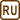## Determination of Metal by Measuring Its Heat Capacity

### Task number: 1275

A sample of unknown metal with a mass of 192 g heated to a temperature of 100 °C was immersed into a brass calorimeter with a mass of 0.128 kg containing 240 g of water at a temperature of 8.4 °C. Provided that the water temperature stabilized at 21.5 °C, calculate the specific heat capacity of the metal and based on this value of capacity identify the metal by using the Handbook of Chemistry and Physics.

Assume that the specific heat capacity of brass is 394 J kg−1K−1.

• #### Hint

Remember, what is the relationship between the heat released by the metal and the heat absorbed by the calorimeter and water?

• #### Analysis

At the beginning the calorimeter and the water had the same temperature. Then we put a sample of unknown metal which had a higher temperature and it started to lose heat and simultaneously warm up both the water and the calorimeter. After some time the temperature stabilized and the calorimeter, water and the piece of metal had the same temperature.

We know that the heat released by a metal sample must equal the total heat absorbed by the calorimeter and water (so-called calorimetric formula). We can easily evaluate the specific heat capacity of the unknown metal from this equation.

• #### Numerical values

 m0 = 0.128 kg the mass of the brass calorimeter m1 = 240 g = 0.24 kg the mass of water t1 = 8.4 °C the initial water temperature m2 = 192 g = 0.192 kg the mass of unknown metal t2 = 100 °C the initial temperature of unknown metal cb = 394 J kg−1K−1 the specific heat capacity of brass tw = 21.5 °C the stabilized water temperature in the calorimeter cm = ? the specific heat capacity of the unknown metal

From the Handbook of Chemistry and Physics:

 cw = 4180 J kg−1K−1 the specific heat capacity of water
• #### Solution

Given that the system is isolated, the heat released by the metal Qr must equal the sum of the heat Qa1 absorbed by water and the heat Qa2 absorbed by the calorimeter (so-called calorimetric formula)

$Q_{\mathrm{r}}=Q_{\mathrm{a1}}+Q_{\mathrm{a2}}.$

According to the definition of specific heat capacity, for each heat apply the following formulas:

Qr = m2 cm (t2 − tw),

Qa1 = m1 cw (tw − t1),

Qa2 = m0 cb (tw − t1),

where cm is the unknown specific heat capacity of the metal, cw is the specific heat capacity of water and cb is the specific heat capacity of brass. By substituting these equations into the calorimetric formula, we get a simple equation

$Q_{\mathrm{r}}=Q_{\mathrm{a1}}+Q_{\mathrm{a2}},$ $m_2c_{\mathrm{m}}\left(t_2-t_{\mathrm{w}}\right)=\left(m_0c_{\mathrm{b}}+m_1c_{\mathrm{w}}\right)\left(t_{\mathrm{w}}-t_1\right),$

from which we can evaluate the specific heat capacity cm we are looking for.

$c_{\mathrm{m}}=\frac{\left(m_0c_{\mathrm{b}}+m_1c_{\mathrm{w}}\right)\left(t_{\mathrm{w}}-t_1\right)}{m_2\left(t_2-t_{\mathrm{w}}\right)}.$

Numerical insertion:

$c_{\mathrm{m}}=\frac{\left(0.128\cdot{394}+0.24\cdot{4180}\right)\cdot\left(21.5-8.4\right)}{0.192\cdot\left(100-21.5\right)}\,\mathrm{J\,kg^{-1}K^{-1}}$ $c_{\mathrm{m}}\dot=916 \,\mathrm{J\,kg^{-1}K^{-1}}$

Comparison with the values listed in the Handbook:

The closest to our result is the value of 900 J kg−1K−1, which is the specific heat capacity of aluminium.

• #### Answer

The specific heat capacity of the unknown metal is approximately 916 J kg−1K−1, which roughly corresponds to the heat capacity of aluminium as listed in the Handbook of Chemistry and Physics.